## Speed Torque Characteristic DC Shunt Motor:

The preceding discussion shows that armature voltage variation gives creeping speeds. The simple rheostatic method provides a Speed Torque Characteristic DC Shunt Motor with little hardness and little stability. Ward Leonard control (smooth variation of voltage), on the other hand, produces a flat characteristic with reasonable hardness and stability, but high initial cost. A simple method with low initial cost, to obtain crawling speeds with sufficient hardness, is depicted in Fig. 1.8. The conventional rheostatic control with a resistance in series with the armature is modified by shunting the armature with a low resistance. By varying the values of series and shunt resistances the speed-torque characteristics can be made to have any desired shape.

In the simple rheostatic control using only a series resistance, the voltage across the armature at no-load is V. The no-load speed is decided by V, whatever be the value of Rs. If the armature is shunted by Rsh the voltage across the armature becomes less than V even at no load. The no-load speed decreases to the desired value with proper values of Rs and Rsh. The smaller the value of Rsh, the lesser is the voltage across the armature at no-load. Eventually, the no-load speed decreases. The value of Rsh is also effective in making the characteristic flat.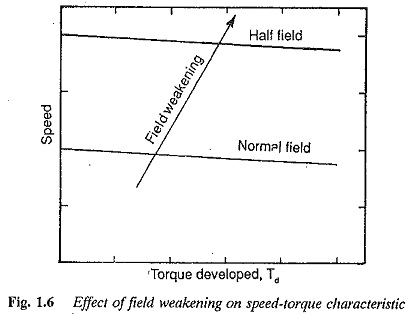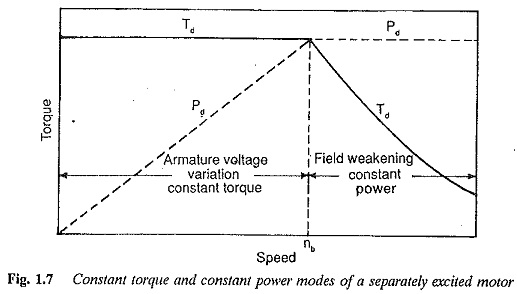Typical speed-torque characteristics are shown in Fig. 1.9 in which the nat­ural characteristic of the DC Shunt Motor and the characteristic with simple rheo­static control are.shown. This modification may be used if stable low speed operation is required. It can be employed for accurate stopping of the drive. By changing the value of Rsh the speed can be reduced to a very low value and then suitable mechanical braking may be applied to have accurate stopping.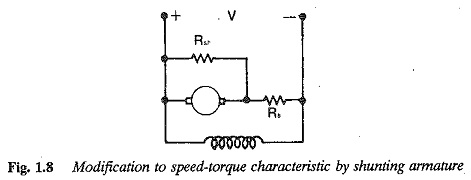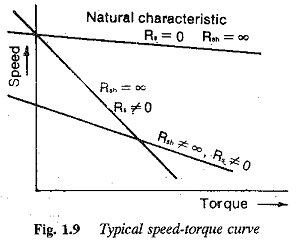Using these equations we have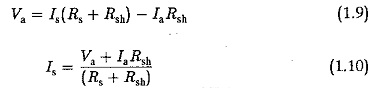Also from Eqs (1.8) and (1.7)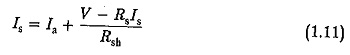Using these relations in Eq. (1.6) we have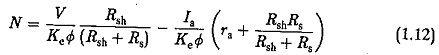Substituting for /a in terms of Td we have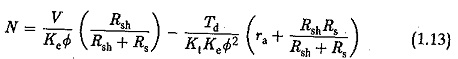The speed-torque characteristic is shown in Fig. 1.9. The following points are clear from the figure:

1.The no load speed (Td = 0) decreases to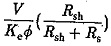Smaller the value of Rsh, smaller is this value. The slope also decreases if Rsh is small. The hardness is thus improved and stable operation is assured when compared to simple rheostatic control.

2.Smoothness of speed control depends on how Rsh and Rs are varied. The speed control is stepped, as the resistances can be varied in a stepped mariner.

3.Speeds below base speed are possible. The no-load speed itself changes following variations in Rsh. A sharp drop in the no-load speed may be observed when Rsh is decreased. Speed control is achieved by varying the value of Rs. The method is equivalent to making the field stronger and gives results similar to those obtained by increasing the field current at a given armature current.

4.The method is suitable for constant torque loads, so that the armature current is at its rated value.

5.The method is suitable if accurate stopping is

6.It is not economical for continuous operation. The losses in Rsh and Rs make the system inefficient. The method can be employed if stable creeping speeds are required for short periods.

Scroll to Top